# RD Sharma Solutions for Class 10 Maths Chapter 7 Statistics Exercise 7.4

The median is the middle of a distribution. In this exercise, students will practice finding the median of a discrete and grouped frequency distribution. For quick access to solutions, the RD Sharma Solutions Class 10 is the best place. It has well-structured solutions in simple language to match the level of all the students. For detailed studies regarding this exercise, download RD Sharma Solutions for Class 10 Maths Chapter 7 Statistics Exercise 7.4 PDF provided below.

## RD Sharma Solutions for Class 10 Maths Chapter 7 Statistics Exercise 7.4 Download PDF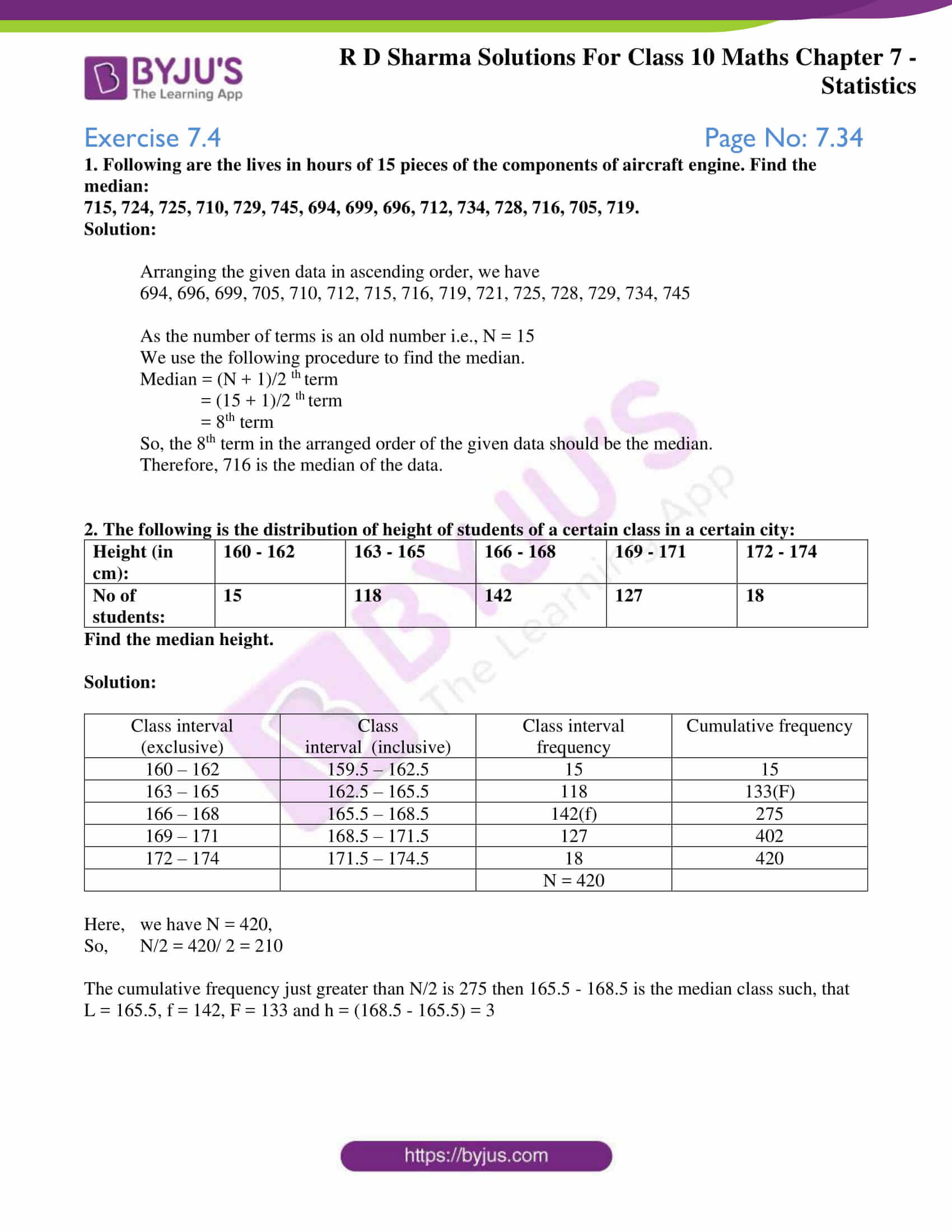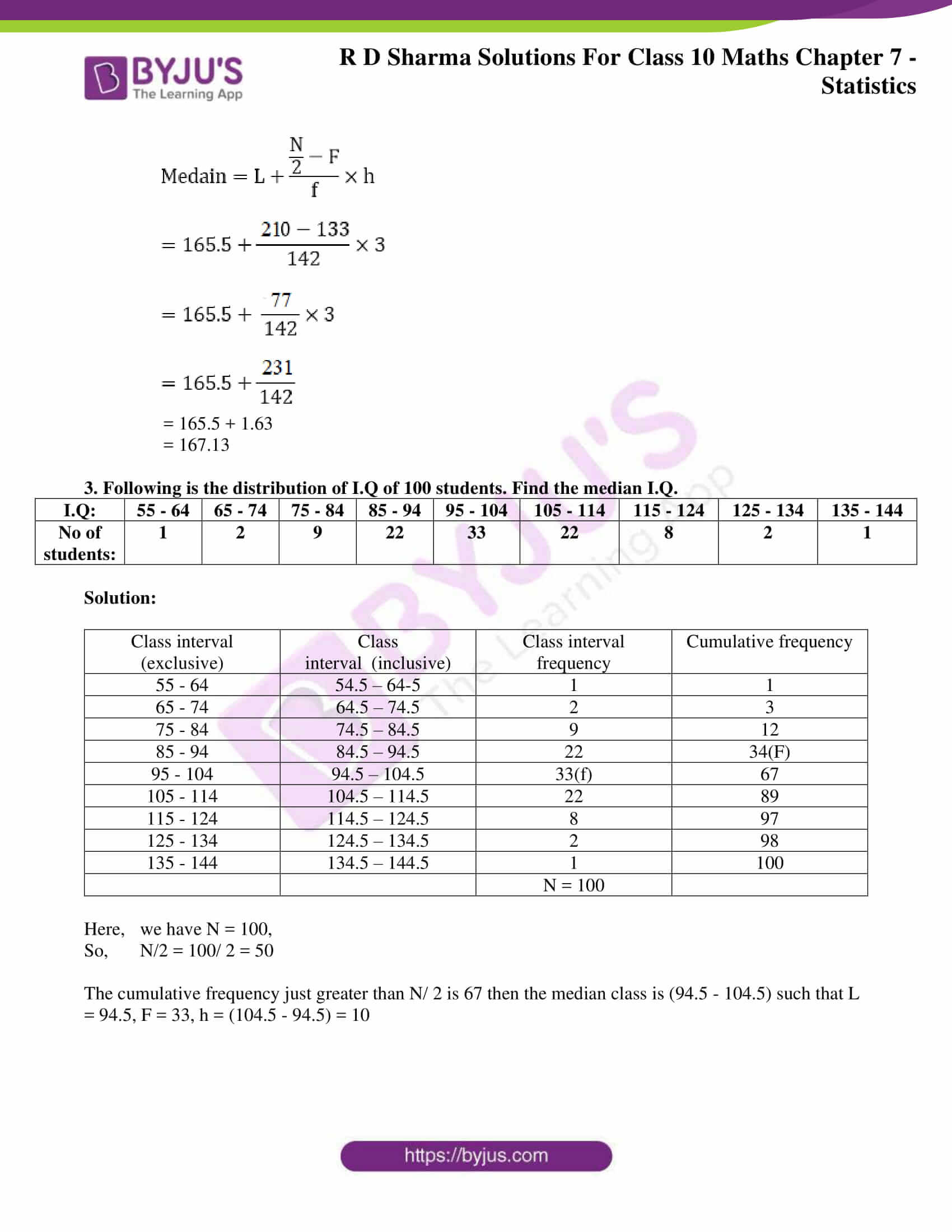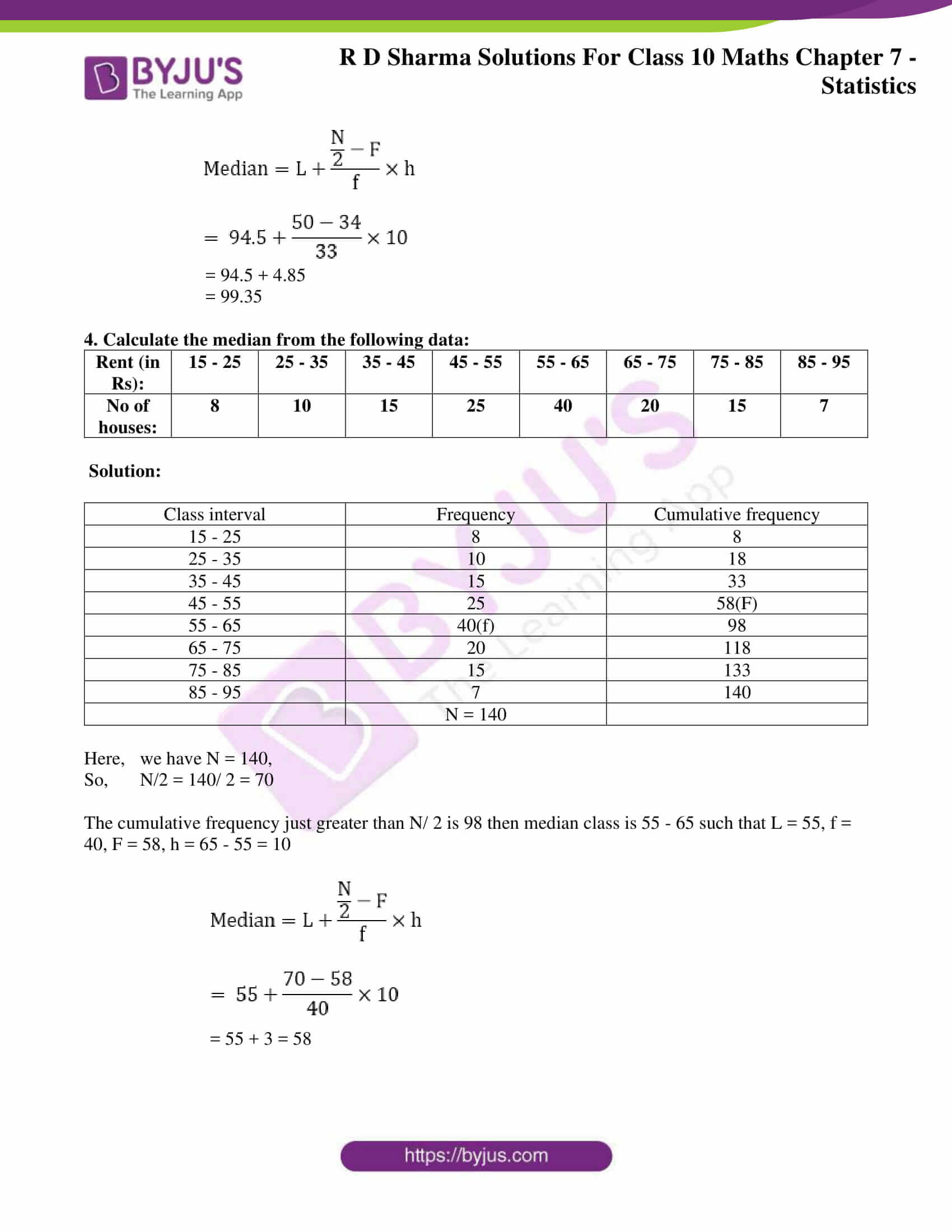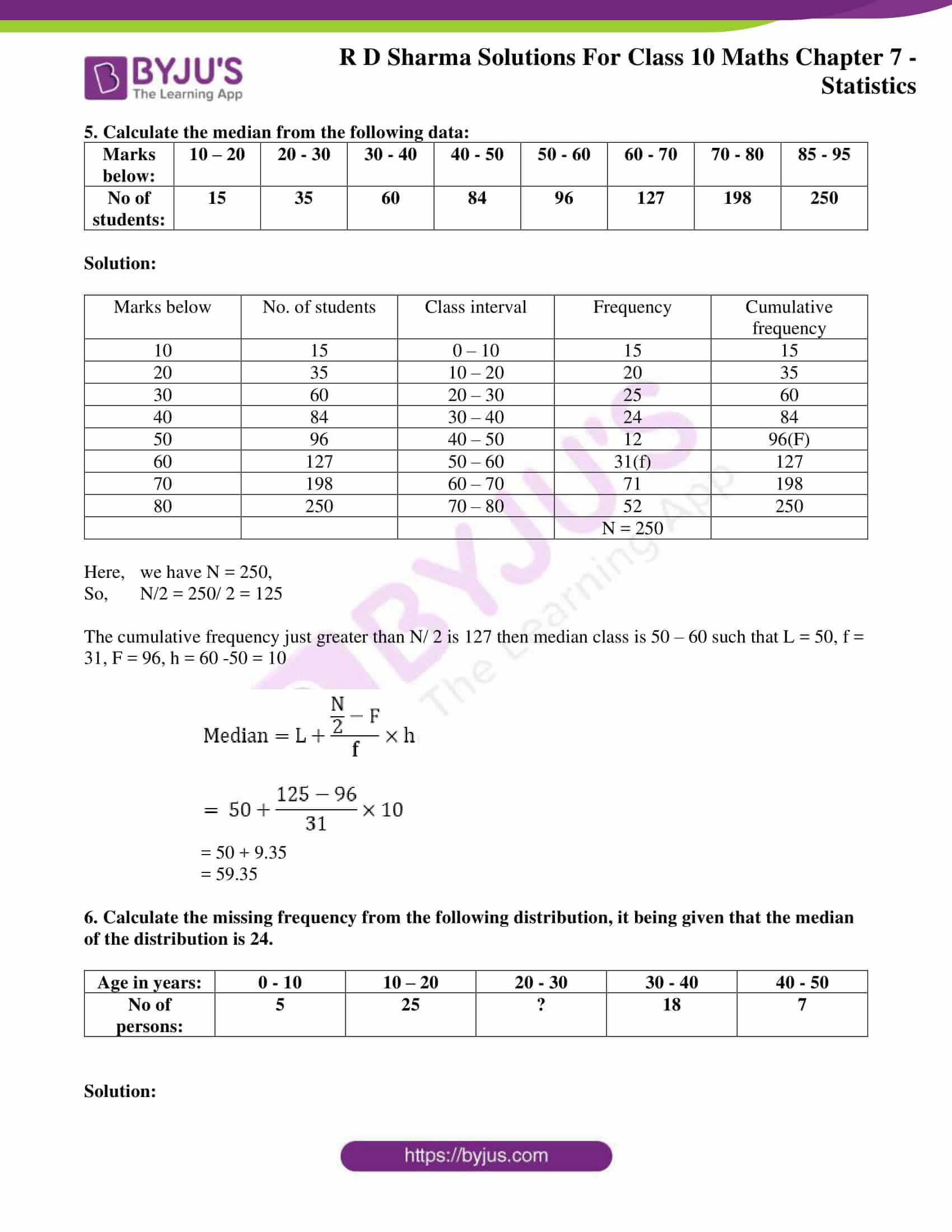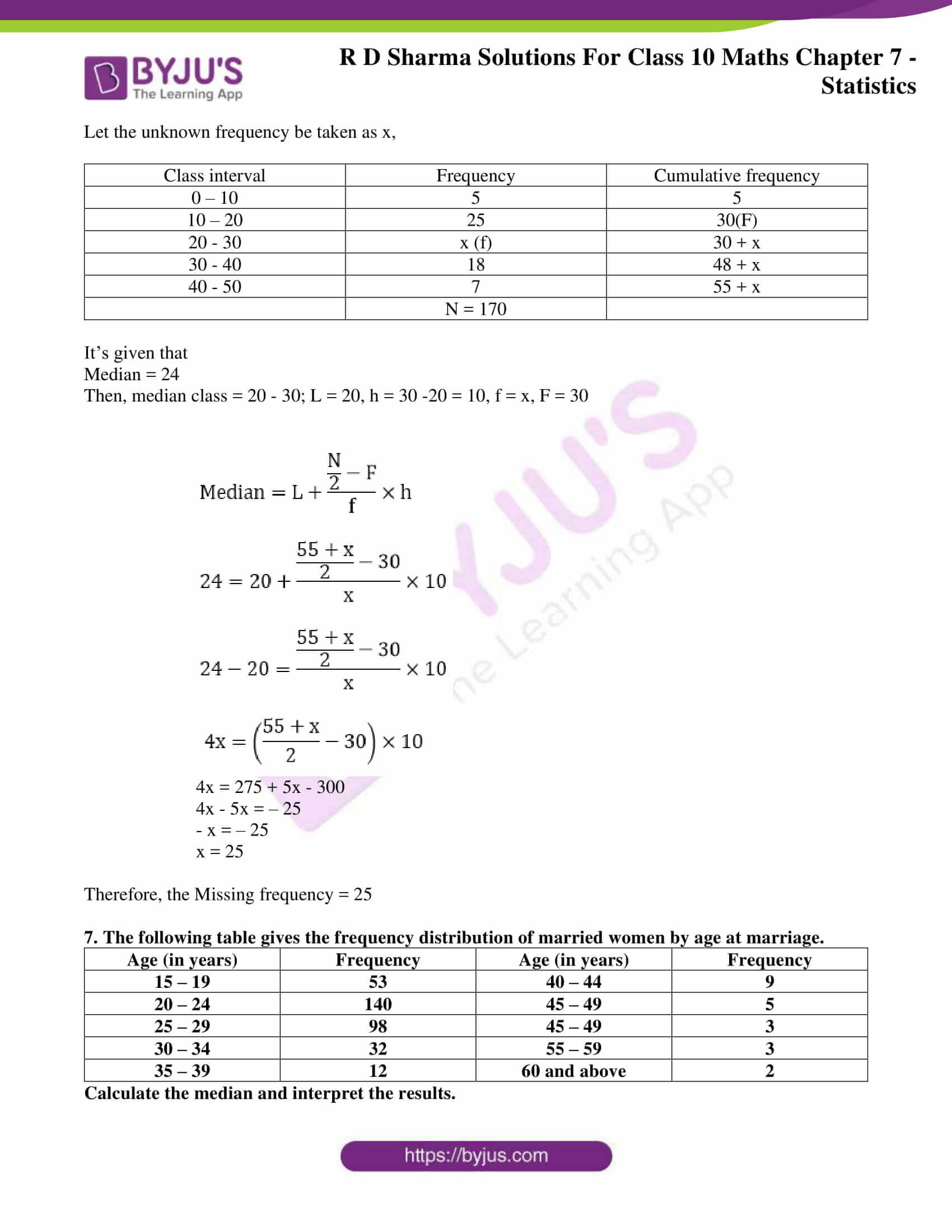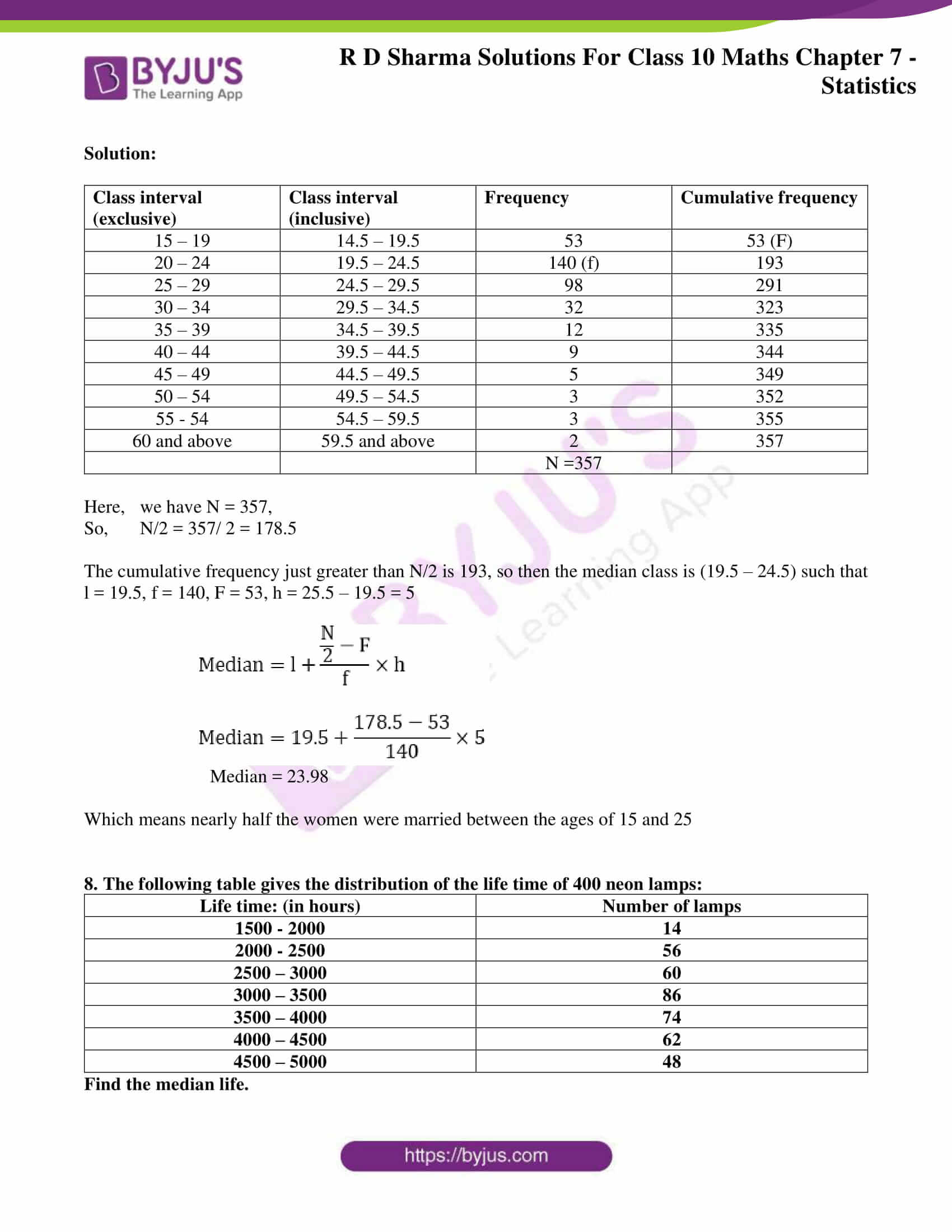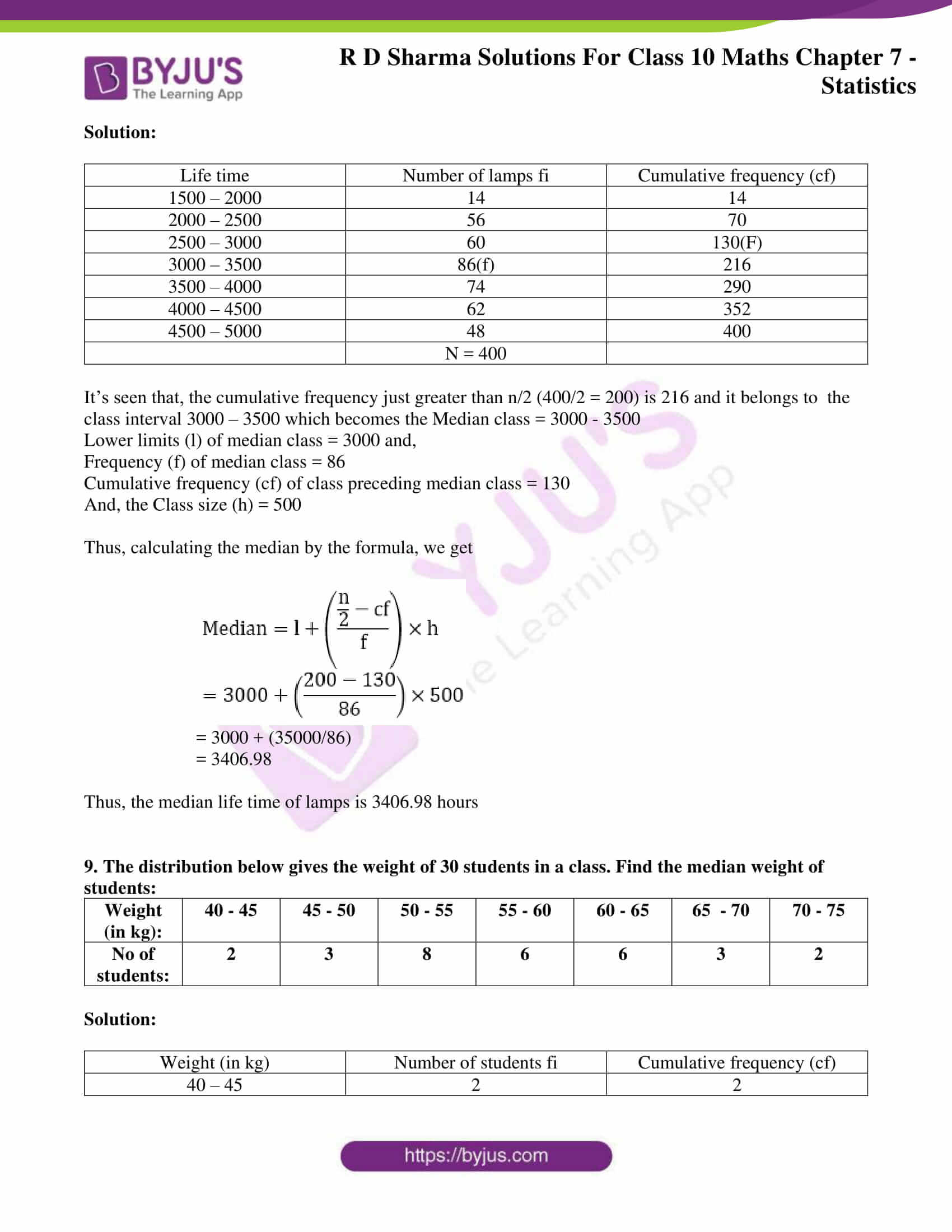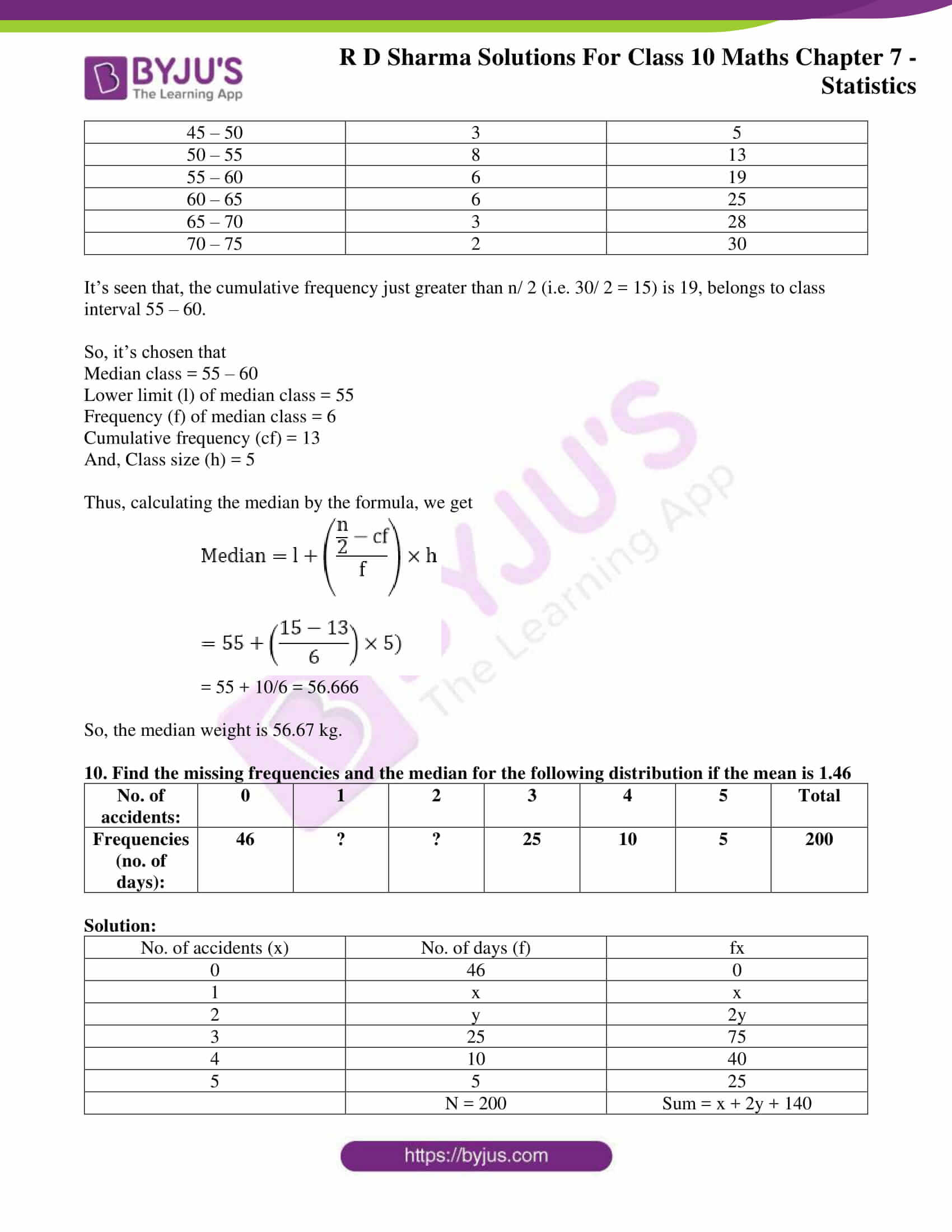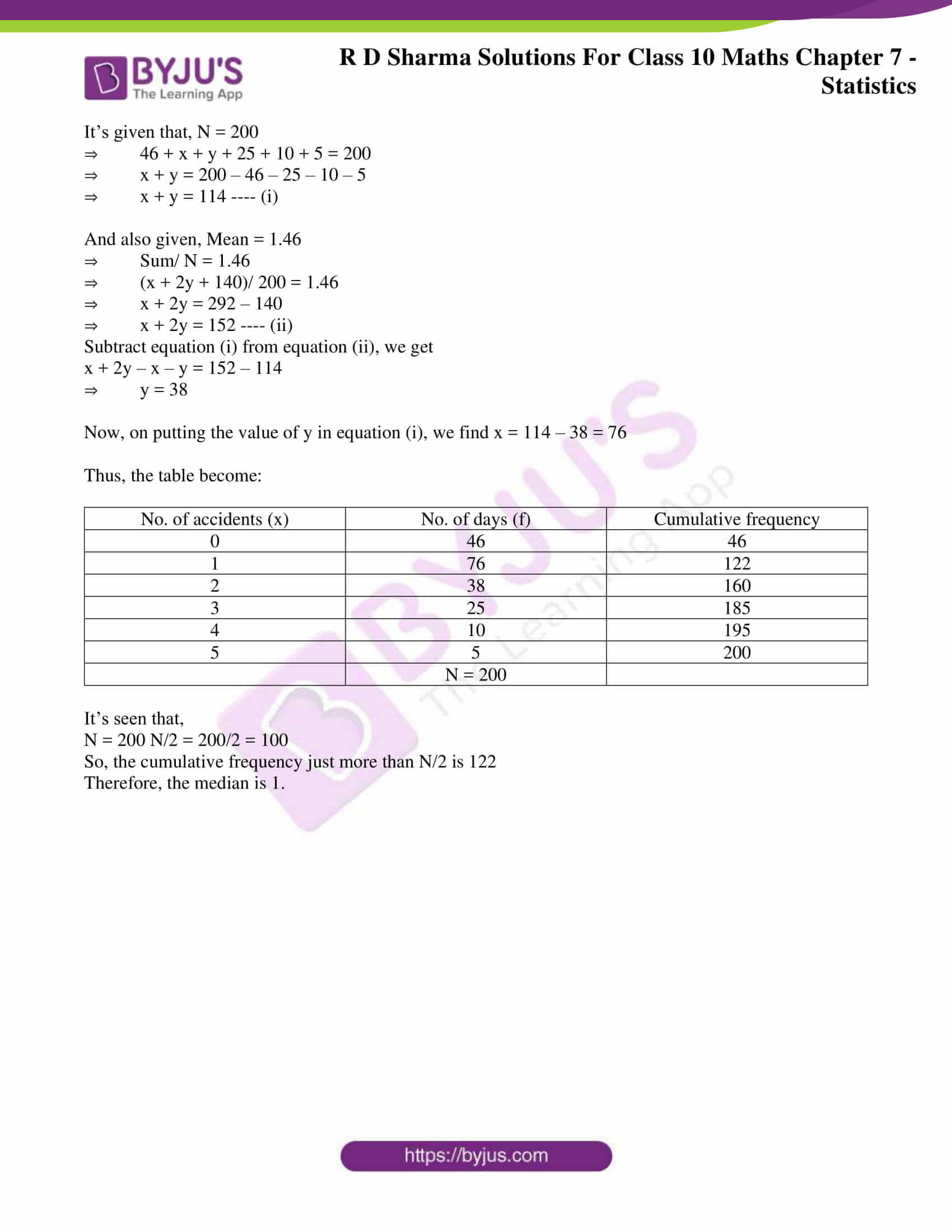### Access RD Sharma Solutions for Class 10 Maths Chapter 7 Statistics Exercise 7.4

1. Following are the lives in hours of 15 pieces of the components of aircraft engine. Find the median:

715, 724, 725, 710, 729, 745, 694, 699, 696, 712, 734, 728, 716, 705, 719.

Solution:

Arranging the given data in ascending order, we have

694, 696, 699, 705, 710, 712, 715, 716, 719, 721, 725, 728, 729, 734, 745

As the number of terms is an old number i.e., N = 15

We use the following procedure to find the median.

Median = (N + 1)/2 th term

= (15 + 1)/2 th term

= 8th term

So, the 8th term in the arranged order of the given data should be the median.

Therefore, 716 is the median of the data.

2. The following is the distribution of height of students of a certain class in a certain city:

 Height (in cm): 160 – 162 163 – 165 166 – 168 169 – 171 172 – 174 No of students: 15 118 142 127 18

Find the median height.

Solution:

 Class interval (exclusive) Class interval  (inclusive) Class interval frequency Cumulative frequency 160 – 162 159.5 – 162.5 15 15 163 – 165 162.5 – 165.5 118 133(F) 166 – 168 165.5 – 168.5 142(f) 275 169 – 171 168.5 – 171.5 127 402 172 – 174 171.5 – 174.5 18 420 N = 420

Here, we have N = 420,

So, N/2 = 420/ 2 = 210

The cumulative frequency just greater than N/2 is 275 then 165.5 – 168.5 is the median class such, that

L = 165.5, f = 142, F = 133 and h = (168.5 – 165.5) = 3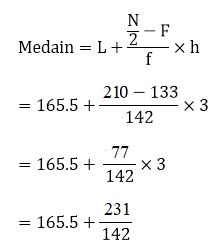= 165.5 + 1.63

= 167.13

3. Following is the distribution of I.Q of 100 students. Find the median I.Q.

 I.Q: 55 – 64 65 – 74 75 – 84 85 – 94 95 – 104 105 – 114 115 – 124 125 – 134 135 – 144 No of students: 1 2 9 22 33 22 8 2 1

Solution:

 Class interval (exclusive) Class interval  (inclusive) Class interval frequency Cumulative frequency 55 – 64 54.5 – 64-5 1 1 65 – 74 64.5 – 74.5 2 3 75 – 84 74.5 – 84.5 9 12 85 – 94 84.5 – 94.5 22 34(F) 95 – 104 94.5 – 104.5 33(f) 67 105 – 114 104.5 – 114.5 22 89 115 – 124 114.5 – 124.5 8 97 125 – 134 124.5 – 134.5 2 98 135 – 144 134.5 – 144.5 1 100 N = 100

Here, we have N = 100,

So, N/2 = 100/ 2 = 50

The cumulative frequency just greater than N/ 2 is 67 then the median class is (94.5 – 104.5) such that L = 94.5, F = 33, h = (104.5 – 94.5) = 10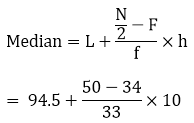= 94.5 + 4.85

= 99.35

4. Calculate the median from the following data:

 Rent (in Rs): 15 – 25 25 – 35 35 – 45 45 – 55 55 – 65 65 – 75 75 – 85 85 – 95 No of houses: 8 10 15 25 40 20 15 7

Solution:

 Class interval Frequency Cumulative frequency 15 – 25 8 8 25 – 35 10 18 35 – 45 15 33 45 – 55 25 58(F) 55 – 65 40(f) 98 65 – 75 20 118 75 – 85 15 133 85 – 95 7 140 N = 140

Here, we have N = 140,

So, N/2 = 140/ 2 = 70

The cumulative frequency just greater than N/ 2 is 98 then median class is 55 – 65 such that L = 55, f = 40, F = 58, h = 65 – 55 = 10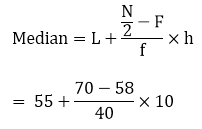= 55 + 3 = 58

5. Calculate the median from the following data:

 Marks below: 10 – 20 20 – 30 30 – 40 40 – 50 50 – 60 60 – 70 70 – 80 85 – 95 No of students: 15 35 60 84 96 127 198 250

Solution:

 Marks below No. of students Class interval Frequency Cumulative frequency 10 15 0 – 10 15 15 20 35 10 – 20 20 35 30 60 20 – 30 25 60 40 84 30 – 40 24 84 50 96 40 – 50 12 96(F) 60 127 50 – 60 31(f) 127 70 198 60 – 70 71 198 80 250 70 – 80 52 250 N = 250

Here, we have N = 250,

So, N/2 = 250/ 2 = 125

The cumulative frequency just greater than N/ 2 is 127 then median class is 50 – 60 such that L = 50, f = 31, F = 96, h = 60 -50 = 10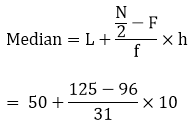= 50 + 9.35

= 59.35

6. Calculate the missing frequency from the following distribution, it being given that the median of the distribution is 24.

 Age in years: 0 – 10 10 – 20 20 – 30 30 – 40 40 – 50 No of persons: 5 25 ? 18 7

Solution:

Let the unknown frequency be taken as x,

 Class interval Frequency Cumulative frequency 0 – 10 5 5 10 – 20 25 30(F) 20 – 30 x (f) 30 + x 30 – 40 18 48 + x 40 – 50 7 55 + x N = 170

It’s given that

Median = 24

Then, median class = 20 – 30; L = 20, h = 30 -20 = 10, f = x, F = 30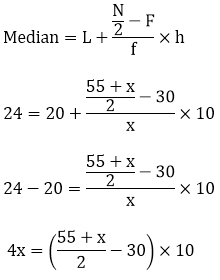4x = 275 + 5x – 300

4x – 5x = – 25

– x = – 25

x = 25

Therefore, the Missing frequency = 25

7. The following table gives the frequency distribution of married women by age at marriage.

 Age (in years) Frequency Age (in years) Frequency 15 – 19 53 40 – 44 9 20 – 24 140 45 – 49 5 25 – 29 98 45 – 49 3 30 – 34 32 55 – 59 3 35 – 39 12 60 and above 2

Calculate the median and interpret the results.

Solution:

 Class interval (exclusive) Class interval (inclusive) Frequency Cumulative frequency 15 – 19 14.5 – 19.5 53 53 (F) 20 – 24 19.5 – 24.5 140 (f) 193 25 – 29 24.5 – 29.5 98 291 30 – 34 29.5 – 34.5 32 323 35 – 39 34.5 – 39.5 12 335 40 – 44 39.5 – 44.5 9 344 45 – 49 44.5 – 49.5 5 349 50 – 54 49.5 – 54.5 3 352 55 – 54 54.5 – 59.5 3 355 60 and above 59.5 and above 2 357 N =357

Here, we have N = 357,

So, N/2 = 357/ 2 = 178.5

The cumulative frequency just greater than N/2 is 193, so then the median class is (19.5 – 24.5) such that l = 19.5, f = 140, F = 53, h = 25.5 – 19.5 = 5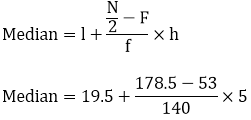Median = 23.98

Which means nearly half the women were married between the ages of 15 and 25

8. The following table gives the distribution of the life time of 400 neon lamps:

 Life time: (in hours) Number of lamps 1500 – 2000 14 2000 – 2500 56 2500 – 3000 60 3000 – 3500 86 3500 – 4000 74 4000 – 4500 62 4500 – 5000 48

Find the median life.

Solution:

 Life time Number of lamps fi Cumulative frequency (cf) 1500 – 2000 14 14 2000 – 2500 56 70 2500 – 3000 60 130(F) 3000 – 3500 86(f) 216 3500 – 4000 74 290 4000 – 4500 62 352 4500 – 5000 48 400 N = 400

It’s seen that, the cumulative frequency just greater than n/2 (400/2 = 200) is 216 and it belongs to the class interval 3000 – 3500 which becomes the Median class = 3000 – 3500

Lower limits (l) of median class = 3000 and,

Frequency (f) of median class = 86

Cumulative frequency (cf) of class preceding median class = 130

And, the Class size (h) = 500

Thus, calculating the median by the formula, we get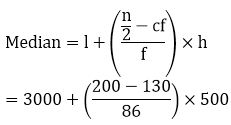= 3000 + (35000/86)

= 3406.98

Thus, the median life time of lamps is 3406.98 hours

9. The distribution below gives the weight of 30 students in a class. Find the median weight of students:

 Weight (in kg): 40 – 45 45 – 50 50 – 55 55 – 60 60 – 65 65 – 70 70 – 75 No of students: 2 3 8 6 6 3 2

Solution:

 Weight (in kg) Number of students fi Cumulative frequency (cf) 40 – 45 2 2 45 – 50 3 5 50 – 55 8 13 55 – 60 6 19 60 – 65 6 25 65 – 70 3 28 70 – 75 2 30

It’s seen that, the cumulative frequency just greater than n/ 2 (i.e. 30/ 2 = 15) is 19, belongs to class interval 55 – 60.

So, it’s chosen that

Median class = 55 – 60

Lower limit (l) of median class = 55

Frequency (f) of median class = 6

Cumulative frequency (cf) = 13

And, Class size (h) = 5

Thus, calculating the median by the formula, we get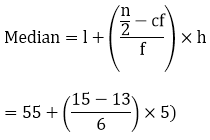= 55 + 10/6 = 56.666

So, the median weight is 56.67 kg.

10. Find the missing frequencies and the median for the following distribution if the mean is 1.46

 No. of accidents: 0 1 2 3 4 5 Total Frequencies (no. of days): 46 ? ? 25 10 5 200

Solution:

 No. of accidents (x) No. of days (f) fx 0 46 0 1 x x 2 y 2y 3 25 75 4 10 40 5 5 25 N = 200 Sum = x + 2y + 140

It’s given that, N = 200

⇒ 46 + x + y + 25 + 10 + 5 = 200

⇒ x + y = 200 – 46 – 25 – 10 – 5

⇒ x + y = 114 —- (i)

And also given, Mean = 1.46

⇒ Sum/ N = 1.46

⇒ (x + 2y + 140)/ 200 = 1.46

⇒ x + 2y = 292 – 140

⇒ x + 2y = 152 —- (ii)

Subtract equation (i) from equation (ii), we get

x + 2y – x – y = 152 – 114

⇒ y = 38

Now, on putting the value of y in equation (i), we find x = 114 – 38 = 76

Thus, the table become:

 No. of accidents (x) No. of days (f) Cumulative frequency 0 46 46 1 76 122 2 38 160 3 25 185 4 10 195 5 5 200 N = 200

It’s seen that,

N = 200 N/2 = 200/2 = 100

So, the cumulative frequency just more than N/2 is 122

Therefore, the median is 1.